Wir verwenden Google für unsere Suche. Mit Klick auf „Suche aktivieren“ aktivieren Sie das Suchfeld und akzeptieren die Nutzungsbedingungen.

# New in SG-Lib 3.9

###### SGTBB(SG)- replaces the complex surface geometry by a simplified bounding box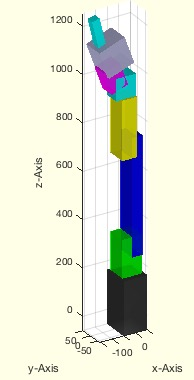% SGTBB(SG) - replaces the complex surface geometry by a simplified bounding box% (by Tim Lueth, VLFL-Lib, 2017-JUN-29 as class: KINEMATICS AND FRAMES)% % Since SG can be very large structures, the call by value concept of % matlab is time consuming. This fnctn replaces the solid geometries by % its bounding box geometries relative to the base frame.% in Contrast to SGofBB or BBofSG this fnctn handles in addition the % frames that are required for cinematic chains (Status of: 2017-07-02)% % Introduced first in SolidGeometry 3.9% % See also: SGTchain, SGTcalibchain, iscollofSG% % SGbb=SGTBB(SG)% === INPUT PARAMETERS ===% SG: cell list of solid geometries% === OUTPUT RESULTS ======% SGbb: cell list of bounding boxes% % See also: SGTchain, SGTcalibchain, iscollofSG%
###### SGTframeChain(nums,extr,B,F)- returns a list of frame connections for a cell list if solids% SGTframeChain(nums,extr,B,F) - returns a list of frame connections for a cell list if solids% (by Tim Lueth, VLFL-Lib, 2017-JUN-26 as class: KINEMATICS AND FRAMES)% % Auxiliary fnctn called from SGTchain. The funxtion can be used to % create a frame-link-table of cell elements. The frame-link-table format % is% [BaseNr FFrame-SG1ind BFrame-SG2ind SG1ind SG2ind rot2nd ztr2nd]% BaseNr: Nr of SG in the final cell list; 0=origin% FFrame: Namestring of first (SG1ind) element's frame % BFrame: Namestring of second (SG2ind) element's frame% SG1ind: Index of first element in Geometry List SGc% SG2ind: Index of second element in Geometry List SGc% rot2nd: z-Rotation wrt to ez of BFrame of second element% ztr2nd: z-translation wrt to ez of BFrame of second element% (Status of: 2017-07-01)% % See also: SGTchain% % [LFrm,LL,Chstr]=SGTframeChain(nums,[extr,B,F])% === INPUT PARAMETERS ===% nums: number, or list of chain elements% extr: optional chaining; default is i-F -> i+1-B% B: extra chaining [SG1ind FFrame SG2ind BFrame] such as [1 'F' 2 'B']% F: Standard Base frame string; default is 'B'% === OUTPUT RESULTS ======% LFrm: Link Frame Tabe% LL: Link list [start index , End index] in SGc% Chstr: compiled string like the extra chaining% % EXAMPLE: % SGTframeChain(6)% SGTframeChain(1:3,'','BX','FX')% SGTframeChain(1:3,[3 'F' 4 'B'],'BX','FX')% SGTframeChain(1:7,[7 'F1' 8 'B' 7 'F2' 8 'B' 7 'F3' 8 'B']);%
###### SGTsetorigin(SG,Nam)- moves the solid into a position that the base frame become the origin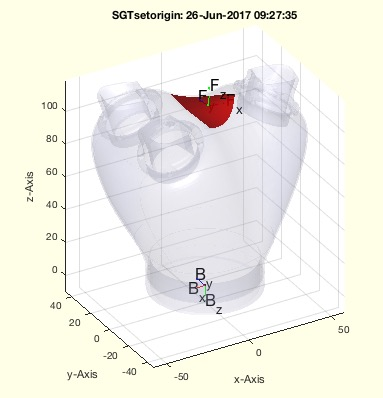% SGTsetorigin(SG,Nam) - moves the solid into a position that the base frame become the origin% (by Tim Lueth, VLFL-Lib, 2017-JUN-26 as class: SURFACES)% % The position of the vertices and of the frames are moved (Status of: % 2017-06-26)% % See also: SGtransT, SGTget% % SGN=SGTsetorigin(SG,Nam)% === INPUT PARAMETERS ===% SG: Solid geometry% Nam: Name of a frame% === OUTPUT RESULTS ======% SGN: spatial transformed SG% % EXAMPLE: % load JACO_robot% SGTsetorigin(JCF,'B')% %
###### SGpuzzlecut3D(SG,bsiz)- creates from a large solid geometry smaller printable parts% SGpuzzlecut3D(SG,bsiz) - creates from a large solid geometry smaller printable parts% (by Tim Lueth, VLFL-Lib, 2017-JUN-25 as class: SURFACES)% % This fnctn helps to separate large Solids in smaller ones for printing % or for half-cuts of technical education (Status of: 2017-06-26)% % See also: SGsurfaceplot, SGwriteSeparatedSTL% % SGs=SGpuzzlecut3D(SG,[bsiz])% === INPUT PARAMETERS ===% SG: Solid Geometry% bsiz: [maxx maxy maxz]; if values are <=1 it is not the size but divider% === OUTPUT RESULTS ======% SGs: List of puzzle elements% % EXAMPLE: % load JACO_robot.mat% SGpuzzlecut3D(JC6,[0.5 0.5 0.5]);% % h=patchofgca; setplotlight(h,'r',1)%
###### isplanarVLFL(VL,FL,thre)- return whether a point cloud or surface is planar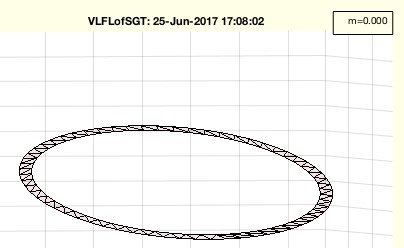% isplanarVLFL(VL,FL,thre) - return whether a point cloud or surface is planar% (by Tim Lueth, VLFL-Lib, 2017-JUN-25 as class: SURFACES)% % works with VL or VL/FL (Status of: 2017-06-25)% % Introduced first in SolidGeometry 3.9% % See also: isconvexPL% % [r,m]=isplanarVLFL(VL,[FL,thre])% === INPUT PARAMETERS ===% VL: Vertex List% FL: optional Facet List to accelerate% thre: Optional threshold; default is 1e-2 ~ 1%% === OUTPUT RESULTS ======% r: true if planar, i.e. m% m: maximal z value / size(BB)% % See also: isconvexPL% % % Copyright 2017 Tim C. Lueth
###### VLFLsurfaceofSGT(SG,N,t,d,s)- returns the flange surface for a given T matrix frame name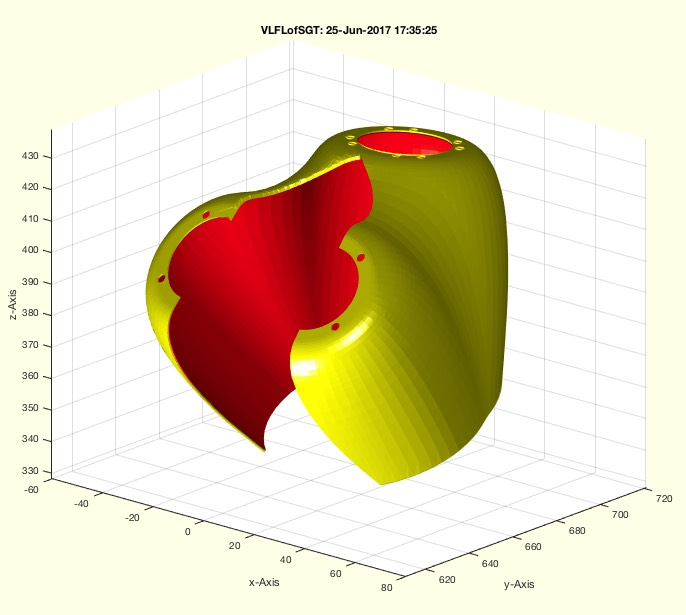% VLFLsurfaceofSGT(SG,N,t,d,s) - returns the flange surface for a given T matrix frame name% (by Tim Lueth, VLFL-Lib, 2017-JUN-25 as class: SURFACES)% % calls fnctn SGofSurface with the surfaces of Frame N (Status of: % 2017-06-25)% % Introduced first in SolidGeometry 3.9% % See also: isplanarVLFL, SGofSurface% % [VL,FL]=VLFLsurfaceofSGT(SG,[N,t,d,s])% === INPUT PARAMETERS ===% SG: Solid Geometry% N: Name of Frame% t: thickness of the solid; default is 0.5% d: distance from the surface; default is 0.3% s: streching of the border line; default is 0% === OUTPUT RESULTS ======% VL: Vertex List% FL: Facet List% % EXAMPLE: % load JACO_robot.mat% X=SGTui(JC01,'T',1);% VLFLsurfaceofSGT(X,'T')% % See also: isplanarVLFL, SGofSurface%
###### NLconformance(NVL,ez);- changes the orientation of a list of normal vectors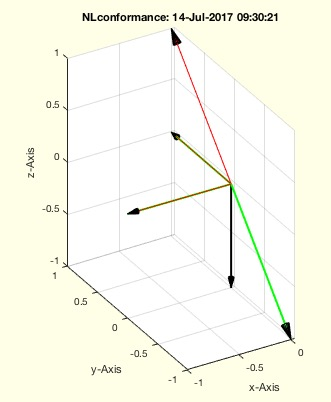% NLconformance(NVL,ez); - changes the orientation of a list of normal vectors% (by Tim Lueth, VLFL-Lib, 2017-JUN-25 as class: ANALYTICAL GEOMETRY)% % in case that a vector has a relative angle of more than 90 degree % (pi/2) to ez, it is turned in direction (multiplied with -1) (Status % of: 2017-06-26)% % Introduced first in SolidGeometry 3.9% % See also: NLcontourVL% % NVL=NLconformance(NVL,ez);% === INPUT PARAMETERS ===% NVL: Normal vector list% ez: reference direction vector.% === OUTPUT RESULTS ======% NVL: Correct normal vector list% % See also: NLcontourVL%
###### cellstrfind(cellstr,str)- returns the position of a string in a cell list of string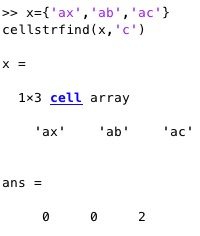% cellstrfind(cellstr,str) - returns the position of a string in a cell list of string% (by Tim Lueth, VLFL-Lib, 2017-JUN-25 as class: AUXILIARY PROCEDURES)% % See also: cell2str, str2cell, cell2array% % f=cellstrfind(cellstr,str)% === INPUT PARAMETERS ===% cellstr: cellstr% str: search string% === OUTPUT RESULTS ======% f: array of position for each cell elememt% % EXAMPLE: % x={'ax','ab','ac'}% cellstrfind(x,'c')%
###### CVLofRL(RL,ez)%- returns a CVL of a radial list and an ez vector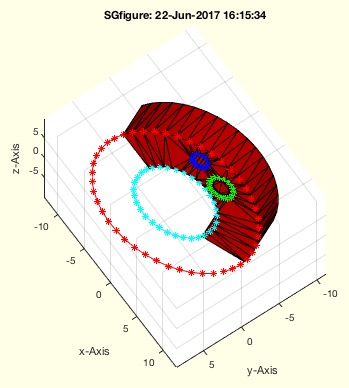% CVLofRL(RL,ez)% - returns a CVL of a radial list and an ez vector% (by Tim Lueth, VLFL-Lib, 2017-JUN-22 as class: ANALYTICAL GEOMETRY)% % This fnctn can be used to postprocess an Radial list of % TR3mountingfaces or CVLdimclassifier% RL has the format:% [xc yc zc r n deg xs ys zs]% [xc yc zc] is the center of the circle% r is the radius% n is the number of found point% deg is the angle of the circle segment% [xs yz zs] can be either a sample point or the norm vector% (Status of: 2017-06-25)% % See also: CVLdimclassifier, TR3mountingfaces, SGTui% % CVL=CVLofRL(RL,[ez])%% === INPUT PARAMETERS ===% RL: Radial list % ez: optional ez vector; or ez vector list list % === OUTPUT RESULTS ======% CVL: Closed Vertex List% % EXAMPLE: % SG=SGtransR(SGsample(25),rot(pi/6,0,pi/2)); % TR3=triangulation(SG.FL,SG.VL);% [a,b,c,d,e]=TR3mountingfaces(TR3,23,1); show% CVL=CVLofRL(e,b); SGfigure; SGplot(SG); CVLplot(CVL);%
###### JACOstruct- creates a global struct JACOs that is used by JACOsim, JACOget, JACOset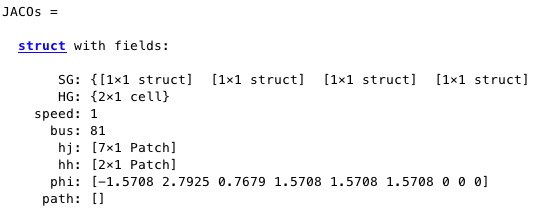% JACOstruct - creates a global struct JACOs that is used by JACOsim, JACOget, JACOset% (by Tim Lueth, ROBOT-Lib, 2017-JUN-20)% % To use the global JACO struct, insert the following line into your % fnctn:% % global JACOs; JACOstruct; % Useage of global JACO robot struct% % It consist of:% JACOs.SG=SGreduceVLFL(JACO,3000); % Geometry of JACO% JACOs.HG={}; % Geometry of obstacles% JACOs.speed=1; % Robot speed% JACOs.bus=81; % Robot USB address% JACOs.hj=[]; % handle for drawing of robot% JACOs.hh=[]; % handle for environment drawing% JACOs.phi=[0 0 0 0 0 0 0 0 0]; % current Position% JACOs.path=[]; % current path% (Status of: 2017-06-21)% % See also: JACOget, JACOset, JACOsim% % JACOstruct% % EXAMPLE: inspect struct and current values% global JACOs; JACOstruct; % Useage of global JACO robot struct% JACOs%
###### opponentFL(FL,fii)- returns the opponents indices for a facet list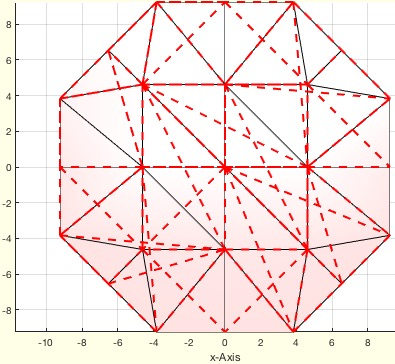% opponentFL(FL,fii) - returns the opponents indices for a facet list% (by Tim Lueth, VLFL-Lib, 2017-JUN-19 as class: SURFACES)% % NOT WELL TESTED YET! (Status of: 2017-06-19)% % OiL=opponentFL(FL,[fii])% === INPUT PARAMETERS ===% FL: Facet list% fii: optional index of facet% === OUTPUT RESULTS ======% OiL: opponent of vertex i in facet fii%
###### JACOset(CMD,PVAL)- sets parameter of the real JACO robot and of the global JACO model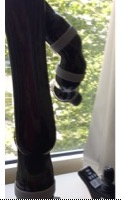% JACOset(CMD,PVAL) - sets parameter of the real JACO robot and of the global JACO model% (by Tim Lueth, ROBOT-Lib, 2017-JUN-19)% % =======================================================================% WORK IN PROGRESS (2017-06-20) - NOT READY FOR FINAL RELEASE % =======================================================================% % See also: JACOget, JACOsim% % [ANSW,t]=JACOset([CMD,PVAL])% === INPUT PARAMETERS ===% CMD: Command string% PVAL: Parameter Values als cell or numeric value% === OUTPUT RESULTS ======% ANSW: % t: %
###### JACOsim(CMD,PVAL)- Simulates the behaviour of the JACO robot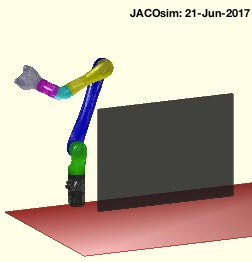% JACOsim(CMD,PVAL) - Simulates the behaviour of the JACO robot% (by Tim Lueth, ROBOT-Lib, 2017-JUN-19)% % This fnctn is used to simulate the behavior of the JACO robot which is % helpful for visualization and collision detection and creating the % programatically interface of JACOget and JACOset and JACOstruc.% JACOsim supports the following commands:% 'init' = sets the JACOstruct to a predefined pose and 'shows' it% 'joints',phi = changes JACOStruct if the pose is collision free% 'show' = plots the pose of the joint values of JACOstruct% 'shows',phi = plots the pose of the given joint values% 'safe',{SG,'under'} = defines safety zones relative to JACO% 'stl', SGs = modifies the kinematic chain/geometry of the robot% JACOsim without an parameter => JACOsim('show') (Status of: 2017-06-21)% % See also: JACOget, JACOset, JACOstruct% % [ANSW,t]=JACOsim([CMD,PVAL])% === INPUT PARAMETERS ===% CMD: command string% PVAL: Parameter Values als cell or numeric value% === OUTPUT RESULTS ======% ANSW: % t: % % EXAMPLE: % JACOsim('init') % Sets the simulation to a initial pose% JACOsim('safe',{A,'alignbottom','infront',-200,'left',0}) % safety zone% JACOsim,('stl',SG) % modifies the kinematic chain/geometry of the robot%
###### nearestcluster(RL,lim)- returns a reduced list of points and a cluster list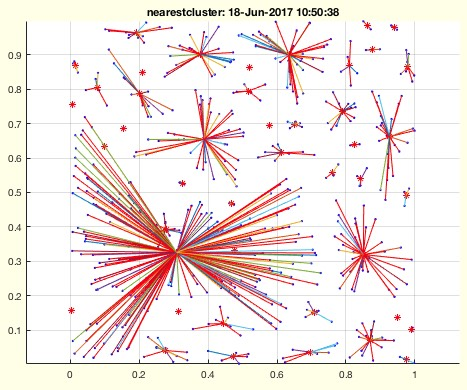% nearestcluster(RL,lim) - returns a reduced list of points and a cluster list% (by Tim Lueth, VLFL-Lib, 2017-JUN-18 as class: ANALYTICAL GEOMETRY)% % Finds cluster of points with a minimal distance of lim;% draws a result only for [nx2 and nx3]% (Status of: 2017-06-18)% % See also: nearestpair, connectofmat% % [RLn,Rn]=nearestcluster(RL,lim)% === INPUT PARAMETERS ===% RL: Point list% lim: maximum distance for clustering% === OUTPUT RESULTS ======% RLn: Points list with replaced mean values% Rn: Cluster matrix related to RL% % EXAMPLE: % [a,b]=nearestcluster(rand(5,2),0.25)% nearestcluster(rand(500,2),0.05);%
###### nofcolmap(col)- returns a number instead of a color string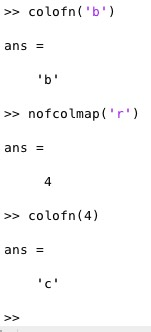% nofcolmap(col) - returns a number instead of a color string% (by Tim Lueth, VLFL-Lib, 2017-JUN-17 as class: USER INTERFACE)% % colmap='kbgcrmyw';% unfortunately, there is a fnctn color of matlab and tim lueth% (Status of: 2017-06-17)% % See also: colofn, color% % col=nofcolmap(col)% === INPUT PARAMETERS ===% col: color integer od string% === OUTPUT RESULTS ======% col: number of color% % EXAMPLE: % colofn('2')% nofcolmap('r')%
###### SGreduceVLFL(SG,f)- returns a solid with reduced% SGreduceVLFL(SG,f) - returns a solid with reduced % (by Tim Lueth, VLFL-Lib, 2017-JUN-17 as class: SURFACES)% % speed optimized; uses reducepatch.% can be used to reduce facet related to the original vertex list (Status % of: 2017-06-17)% % See also: reducepatch, findVL% % [SG,FL,vi]=SGreduceVLFL(SG,[f])% === INPUT PARAMETERS ===% SG: Original Solid Geometry% f: reduction factor or number of facets per% === OUTPUT RESULTS ======% SG: SG with reduced vertices and facets% FL: Facet List related to original solid geometry (slows down by findVL)% vi: index of new vertices related to original solid geometry% % EXAMPLE: % a=SGsample(17); a=SGstripfields(a)% SGreduceVLFL(a,800)% SGreduceVLFL(a,0.5)% [~,FL]=SGreduceVLFL(a,0.1), VLFLplot(a.VL,FL)%
###### sofBB(SG,minv)- returns the maximal dimension of the bounding box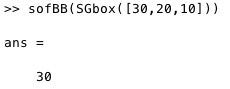% sofBB(SG,minv) - returns the maximal dimension of the bounding box% (by Tim Lueth, VLFL-Lib, 2017-JUN-17 as class: AUXILIARY PROCEDURES)% % Useful fnctn for some SG plot fnctn (Status of: 2017-06-17)% % See also: sizeVL% % [ms,s]=sofBB(SG,[minv])% === INPUT PARAMETERS ===% SG: VL oder SG% minv: optional minimal value; default is 0% === OUTPUT RESULTS ======% ms: max dimension% s: [sx,sy,sz]%
###### setplotlight(hi,col,alpha);- changes the alpha value of a given graphics object handle% setplotlight(hi,col,alpha); - changes the alpha value of a given graphics object handle% (by Tim Lueth, VLFL-Lib, 2017-JUN-16 as class: VISUALIZATION)% % Similar to VLFLplotlight, but sets the parameter individually for a % patch handle (Status of: 2017-06-17)% % See also: VLFLplotlight% % setplotlight(hi,[col,alpha]);% === INPUT PARAMETERS ===% hi: patch handle% col: color value or letter; optional% alpha: alpha index% % EXAMPLE: % SGfigure; [~,~,h]=SGsurfaceplot(SGsample(20)); view(-30,30);% setplotlight(h,'',0.4); show% setplotlight(h,'b',0.4); show% % %
###### rcmod1(n,cx,sq)- return rows and colum for a given number and col length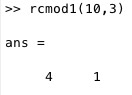% rcmod1(n,cx,sq) - return rows and colum for a given number and col length% (by Tim Lueth, VLFL-Lib, 2017-JUN-16 as class: AUXILIARY PROCEDURES)% % Useful for subplot indi (Status of: 2017-06-16)% % See also: mod1, modn% % rc=rcmod1(n,cx,[sq])% === INPUT PARAMETERS ===% n: index% cx: number of cols% sq: if true; cx is the length of a squared field; default is false% === OUTPUT RESULTS ======% rc: [row col] =[1... 1...]%
###### SGTcalibchain(SGs,phi,Fram)- changes the base frames of a set of solid geoemtries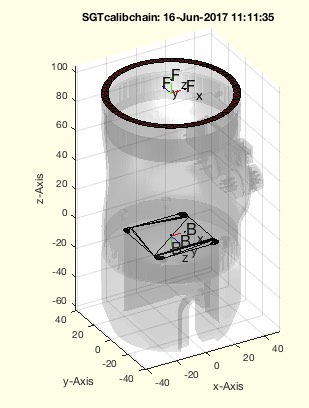% SGTcalibchain(SGs,phi,Fram) - changes the base frames of a set of solid geoemtries% (by Tim Lueth, VLFL-Lib, 2017-JUN-16 as class: KINEMATICS AND FRAMES)% % This fnctn is useful if frames such as base frame or follower frame % were originally created manually from the STL files using SGTui but the % real frames have other orientations. % In this case, the fnctn SGTchain shows only the right pose for a set of % angles if an angle offset if added. If this offset angle is known, the % real configuration can be shown by SGTchain(offset) using the offset % angles.% To avoid the offset angle it is possible to calibrate the z axis of the % Base frame or the follower frame. (Status of: 2017-06-16)% % See also: SGTchain% % SGn=SGTcalibchain(SGs,[phi,Fram])% === INPUT PARAMETERS ===% SGs: Set of solids, with base and folloer frame% phi: offset angle for the % Fram: Frame name; default is 'B'% === OUTPUT RESULTS ======% SGn: Set of solids, with turned frames% % EXAMPLE: % load JACO_robot.mat% SGTchain(JACO,[[0 0 -90 +180 +90 180 180]]/180*pi); view(-160,0); % % shows the zeros pose using an offset% X=SGTcalibchain(JACO,[[0 0 -90 +180 +90 180 180]]/180*pi) % turns the % base frames% SGTchain(X,[0 0 0 0 0 0 0])% SGTchain(X,[0 0 180 180 0 0 0]/180*pi); view(-160,0)%
###### iscollofSG(SGA,SGB,full,firstc,shape);- calculate collision vertices of two solids or a solid chain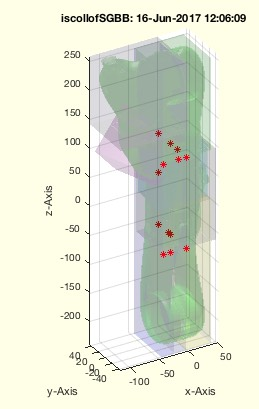% iscollofSG(SGA,SGB,full,firstc,shape); - calculate collision vertices of two solids or a solid chain% (by Tim Lueth, VLFL-Lib, 2017-JUN-14 as class: KINEMATICS AND FRAMES)% % If result is empty; there is no collision% In case of (Status of: 2017-06-20)% % See also: SGreduceVLFL, VLcrossingSG% % res=iscollofSG(SGA,[SGB,full,firstc,shape]);% === INPUT PARAMETERS ===% SGA: Solid A% SGB: Solid B; if empty SGA is used% full: true=full FL; false=Bounding box; default is false% firstc: true = stops after first crossings; false=complete; default=true% shape: if true; bounding boxe is relative to base frame; default=true% === OUTPUT RESULTS ======% res: List of crossing points%
###### isSG(SG)- return whether an object is a Solid Geometry% isSG(SG) - return whether an object is a Solid Geometry% (by Tim Lueth, VLFL-Lib, 2017-JUN-14 as class: SURFACES)% % See also: isconvexPL, isPL% % res=isSG(SG)% === INPUT PARAMETERS ===% SG: Object% === OUTPUT RESULTS ======% res: result% % EXAMPLE: % isSG(PLcircle(4))% isSG(SGofCPLz(PLcircle(4),2))%
###### SGTchain(SGs,phi,z,nums,extr,B,F)- returns the spatial transformed solids of a kinematic chain% SGTchain(SGs,phi,z,nums,extr,B,F) - returns the spatial transformed solids of a kinematic chain % (by Tim Lueth, VLFL-Lib, 2017-JUN-14 as class: KINEMATICS AND FRAMES)% % If no extra parameters are used, all connections are done using 'B' % Base frame and 'F' Follower Frame% The first rotation is currently ignored and can be called using NaN or % zeros. i.e. the base frame of the first element is always unchanged.% The parameter nums, extr, B and F are identical to SGTframechain to % define a the frame chain% for improved reaing and debugging it is possible to create a frame % chain once and use it as parameter nums (Status of: 2017-07-01)% % See also: SGTframeChain, SGTBB, SGTcalibchain, SGTplot, SGTui% % SGn=SGTchain(SGs,[phi,z,nums,extr,B,F])% === INPUT PARAMETERS ===% SGs: Cell list of n Solid Geometries% phi: vector of length n of radial rotations around the F-z frame% z: optional z value in ez direction % nums: optional chaining; nums can also be SGTframechain% extr: extra chaining [SG1ind FFrame SG2ind BFrame] such as [1 'F' 2 'B']% B: Standard Base frame string; default is 'B'% F: Standard follower frame string; default is 'F'% === OUTPUT RESULTS ======% SGn: Cell list of n spatial transformed Solid Geometries% % EXAMPLE: % load ('JACO_robot')% JACO={JC0,JC1,JC2,JC3,JC4,JC5,JC6}% SGfigure; SGplot(JACO); view(-30,30); show% SGTchain(JACO,[NaN pi/2]);% SGTchain(JACO,[NaN pi/1 pi/2 pi/3 pi/4 pi/5 pi/6]);% % a=JACOget('joints',1:6)% SGTchain(JACO,[+[NaN a]+[0 0 -90 +180 +90 180 180]]/180*pi); % view(-160,0)% ad=[-90 160 44 90 90 90]/180*pi; SGTchain(JACO,[NaN ad]); view(-160,0);% % Fchain=SGTframeChain(1:7,[7 'F' 8 'B' 7 'F2' 8 'B' 7 'F3' 8 'B'])% SGTchain(JACO,[NaN pi/2 pi pi],+100,Fchain)% % SGTchain([JACO,{JCF}],'','',false,1:7,[7 'F1' 8 'B' 7 'F2' 8 'B' 7 'F3' % 8 'B'])%
###### JACOget(CMD,PVAL)- returns information on the JACO robot by direct calls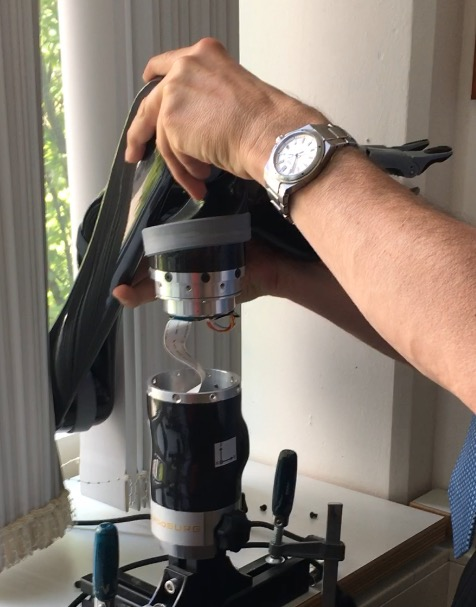% JACOget(CMD,PVAL) - returns information on the JACO robot by direct calls% (by Tim Lueth & Samuel Detzel, ROBOT-Lib, 2017-JUN-13)% % =======================================================================% WORK IN PROGRESS (2017-06-20) - NOT READY FOR FINAL RELEASE % =======================================================================% % Commands are processed sequentially. % Therefor, if a specific jaco is called, use:% JACOget('jaco',2,'joints',1:6) to get the first six joints of jaco% Current commands are:% 'jaco' = number of jaco = [1...16]% 'joints' = get joint values = [1:9] (Status of: 2017-06-13)% % See also: PEAKinstall, JACOset, JACOsim% % [ANSW,t]=JACOget([CMD,PVAL])% === INPUT PARAMETERS ===% CMD: Command string% PVAL: Numeric Parameter Values% === OUTPUT RESULTS ======% ANSW: Answer % t: time delay between call and answer% % EXAMPLE: Get information on the current joints of jaco #1% JACOget('joints',1:3)% JACOget('jaco',2,'joints',1:9,'jaco',1,'joints',1:4)%
###### SGof2CPLzbranch(CPLA,CPLB,z,stype,ctype)- Creates a Solid for an 1:n or n:1 solid branch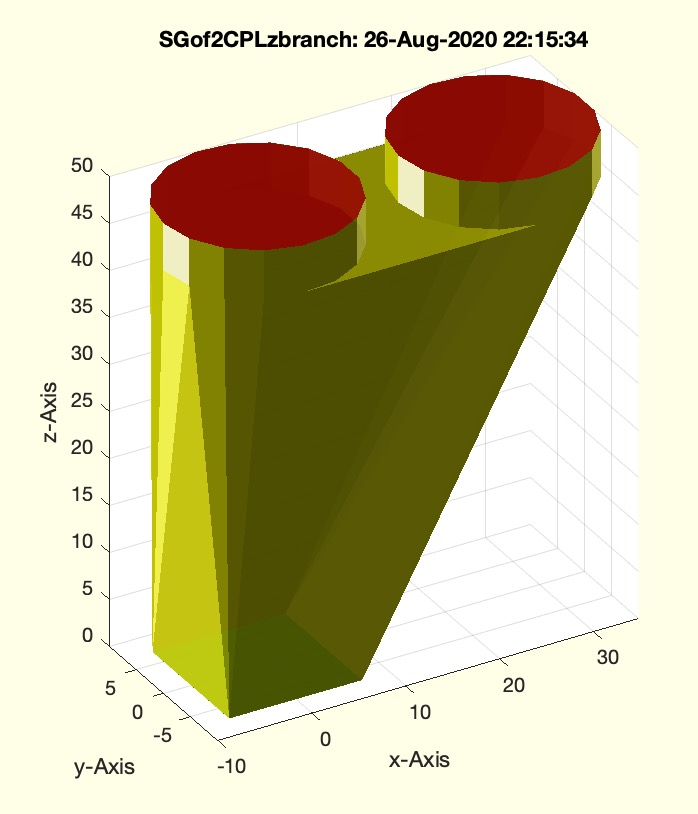% SGof2CPLzbranch(CPLA,CPLB,z,stype,ctype) - Creates a Solid for an 1:n or n:1 solid branch% (by Tim Lueth, VLFL-Lib, 2017-JUN-12 as class: SURFACES)% % Creates a 1:n or n:1 solid branch.% Either CPLA or CPLB must consist of ONLY ONE contour (Status of: % 2017-06-13)% % See also: SGof2CPLz, SGof2CPLsz, SGof2CPLzheurist% % [SG,NFLW,NFLA,NFLB]=SGof2CPLzbranch([CPLA,CPLB,z,stype,ctype])% === INPUT PARAMETERS ===% CPLA: Contour A% CPLB: Contour B% z: height% stype: "number", "length", "angle", "center"; default is "length"% ctype: "mina" or "miny"; default is "mina"% % === OUTPUT RESULTS ======% SG: Solid Geometry of the branch% NFLW: Wall Facets% NFLA: Bottom Facets% NFLB: Cover Facets% % EXAMPLE: % SGof2CPLzbranch(CPLsample(2), CPLsample(9),50)% SGof2CPLzbranch(CPLsample(6), CPLsample(10),50)%
###### SGof2CPLzheurist(CPLA,CPLB,z,stype,ctype)- EXPERIMENT to show the connection of two contours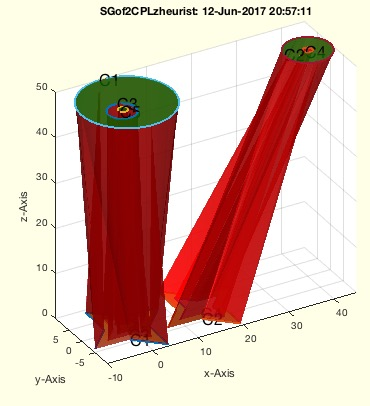% SGof2CPLzheurist(CPLA,CPLB,z,stype,ctype) - EXPERIMENT to show the connection of two contours % (by Tim Lueth, VLFL-Lib, 2017-JUN-11 as class: SURFACES)% % This fnctn is the same as SGof2CPLsz but supports more than one % contour! The optimal result depends on the expectations of the user. % Consider different options for stype carefully: % "number" of points, i.e. 1:nmax % "length" of contour, i.e. 0:sum(edge length) % "angle" of contour, i.e. 0:sum(abs(edge angle)) % "center" of contour, i.e. 0:360 degree % also the starting point ctype ('rot' or 'min'); % Quite optimal procedure to find the walls between two planar contours % without adding new points. The number of faces is na+nb. % The resulting vertex list SG: % SG.VL=[VLaddz(CPA,0);VLaddz(CPB,z)]; % SG.FL=[FLA;FLB;FLW];% (Status of: 2017-06-12)% % See also: SGof2CPLz, SGof2CPLsz, SGof2CPLzbranch, SGanalyzeGroupParts, % SGseparate, SGanalyzePenetration% % [SG,NFW,FLA,FLB,CC,LA,LB]=SGof2CPLzheurist([CPLA,CPLB,z,stype,ctype])% === INPUT PARAMETERS ===% CPLA: Contour A% CPLB: Contour B% z: height z% stype: "number", "length", "angle", "center"; default is "length"% ctype: "mina" or "miny"; default is "mina"% === OUTPUT RESULTS ======% SG: New Vertex List% NFW: New Wall List% FLA: New Bottom FL% FLB: New TOP FL% CC: Correspondance list% LA: Index of Contours A in NVL% LB: Index of Contours B in NVL% % EXAMPLE: % SGof2CPLzheurist(CPLsample(14), CPLsample(26),'','angle');%
###### ELofCIL(CIL,cl)- Converts a Contour Index List into an Edge list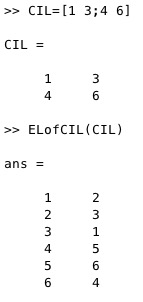% ELofCIL(CIL,cl) - Converts a Contour Index List into an Edge list% (by Tim Lueth, VLFL-Lib, 2017-JUN-11 as class: AUXILIARY PROCEDURES)% % Contour index list [start index end index; start index end index ,...]% (Status of: 2017-06-11)% % See also: ELofn, CILofEL% % EL=ELofCIL(CIL,[cl])% === INPUT PARAMETERS ===% CIL: Contour index list [start index end index; start end ,...]% cl: closing; default is true% === OUTPUT RESULTS ======% EL: Edge List%
###### delaunayofCPL(CPLB)- more sharp delaunay triangulation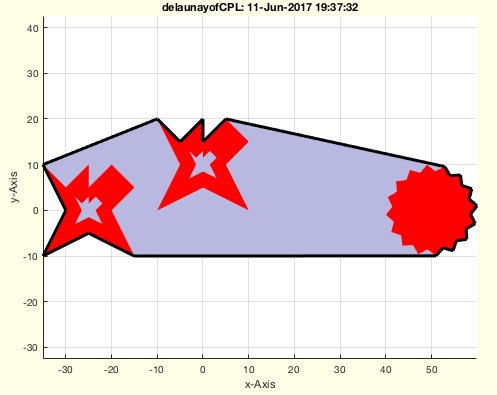% delaunayofCPL(CPLB) - more sharp delaunay triangulation% (by Tim Lueth, VLFL-Lib, 2017-JUN-11 as class: CLOSED POLYGON LISTS)% % The delaunayTriangulation fnctn an be used to create a convex enclosing % facet. On the other hand, this convex contour also includes facets, % which are not necessary to enclose the original contours.% The fnctn also provides the edge list of the constricting contour. % (Status of: 2017-06-11)% % See also: delaunatTriangulation% % [TR3,oi,nfi,fi,EL]=delaunayofCPL(CPLB)% === INPUT PARAMETERS ===% CPLB: Closed Contour List% === OUTPUT RESULTS ======% TR3: delaunayTriangulation% oi: removed facets from outside% nfi: outside facets% fi: fi=isInterior(TR3)% % EL: Edge List of the % % EXAMPLE: % CPLB=[CPLsample(3)+[-25 0];NaN NaN;PLstar(10)+[0 10];NaN % NaN;PLstar(10)+[50 0]];%
###### VLofCVL(CVL)- creates a Contour index list for a CPL/CVL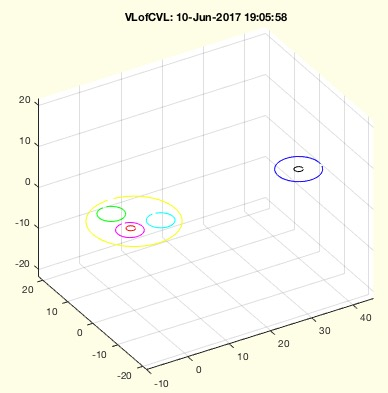% VLofCVL(CVL) - creates a Contour index list for a CPL/CVL% (by Tim Lueth, VLFL-Lib, 2017-JUN-10 as class: CLOSED POLYGON LISTS)% % This is in fact the same fnctn as CILofCVL which is called in this % fnctn.% (Status of: 2017-06-10)% % Introduced first in SolidGeometry 3.9% % See also: CVLofVLCIL% % [VL,CIL,EL]=VLofCVL(CVL)% === INPUT PARAMETERS ===% CVL: CLosed Polygon list CPL/CVL% === OUTPUT RESULTS ======% VL: Vertex list without doubles and NaNs% CIL: Contour index list related to VL% EL: % % EXAMPLE: % [VL,CIL]=VLofCVL(CPLsample(26))% % See also: CVLofVLCIL%
###### CILofCVL(CPL)- creates a Contour index list for a CPL/CVL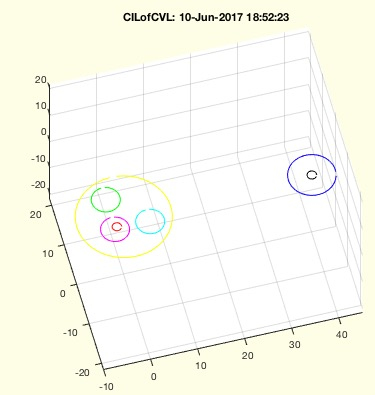% CILofCVL(CPL) - creates a Contour index list for a CPL/CVL% (by Tim Lueth, VLFL-Lib, 2017-JUN-10 as class: CLOSED POLYGON LISTS)% % [CILC,VL,CIL]=CILofCVL(CPL)% === INPUT PARAMETERS ===% CPL: CLosed Polygon list CPL/CVL% === OUTPUT RESULTS ======% CILC: Contour index list related to CPL% VL: Vertex list without doubles and NaNs% CIL: Contour index list related to VL% % EXAMPLE: % [CILC,VL,CIL]=CILofCVL(CPLsample(26))%
###### cellofNaN(CVL)- converts a CVL/CPL into a cell list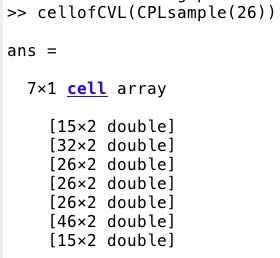% cellofNaN(CVL) - converts a CVL/CPL into a cell list% (by Tim Lueth, VLFL-Lib, 2017-JUN-10 as class: AUXILIARY PROCEDURES)% % See also: selectNaN, separateNaN, replaceNaN% % VLcell=cellofNaN(CVL)% === INPUT PARAMETERS ===% CVL: CVL/CPL separated by NaN% === OUTPUT RESULTS ======% VLcell: Cell list of separated PL/CVL% % EXAMPLE: % cellofNaN(CPLsample(26))% cellofNaN(CPLsample(27))%
###### CVLofVLCIL(VL,CIL)- returns a CPL/CVL from a PL or VL using a contour index list% CVLofVLCIL(VL,CIL) - returns a CPL/CVL from a PL or VL using a contour index list% (by Tim Lueth, VLFL-Lib, 2017-JUN-09 as class: CLOSED POLYGON LISTS)% % CIL= [startindex endindex; startindex endindex; ]% (Status of: 2017-06-09)% % See also: PLofCPL, CPLofPL, CILofCVL% % CVL=CVLofVLCIL(VL,CIL)% === INPUT PARAMETERS ===% VL: Point or Vertex list% CIL: [startindex endindex; startindex endindex; ]% === OUTPUT RESULTS ======% CVL: CLosed Polygon List or Closed Vertex List% % EXAMPLE: % VL=rand(6,3)% CVLofVLCIL(VL,[1 3;4 6])%
###### reversesortindex(iO)- returns the reverse sort index for a sort index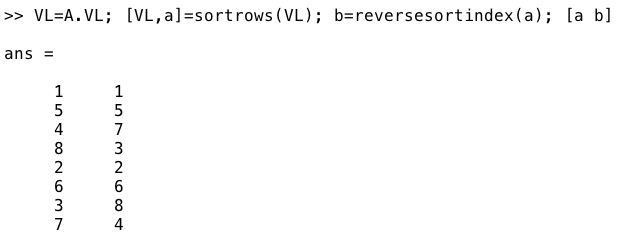% reversesortindex(iO) - returns the reverse sort index for a sort index% (by Tim Lueth, VLFL-Lib, 2017-JUN-09 as class: AUXILIARY PROCEDURES)% % rO=sortrows([iO [1:size(iO)]']); rO=rO(:,2); (Status of: 2017-06-09)% % See also: maprows, findVL% % rO=reversesortindex(iO)% === INPUT PARAMETERS ===% iO: result of sortrows% === OUTPUT RESULTS ======% rO: reverse sort order% % EXAMPLE: % A=SGbox([30 20 10]);% VL=A.VL, [VL,a]=sortrows(VL), b=reversesortindex(a)% SGfigure; VLFLplot(VL,b(FL),'y');%
###### exp_2017_06_07(CPLA,CPLB,z)- EXPERIMENT to show the connection of two contours => SGof2CPLzheurist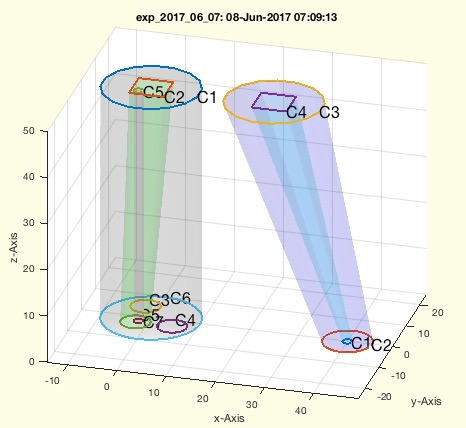% exp_2017_06_07(CPLA,CPLB,z) - EXPERIMENT to show the connection of two contours => SGof2CPLzheurist% (by Tim Lueth, VLFL-Lib, 2017-JUN-08 as class: EXPERIMENTS)% % This fnctn is the same as SGof2CPLz but supports more than one contour! % The optimal result depends on the expectations of the user. Consider % different options for stype carefully: % "number" of points, i.e. 1:nmax % "length" of contour, i.e. 0:sum(edge length) % "angle" of contour, i.e. 0:sum(abs(edge angle)) % "center" of contour, i.e. 0:360 degree % also the starting point ctype ('rot' or 'min'); % Quite optimal procedure to find the walls between two planar contours % without adding new points. The number of faces is na+nb. % The resulting vertex list SG: % SG.VL=[VLaddz(CPA,0);VLaddz(CPB,z)]; % SG.FL=[FLA;FLB;FLW];% (Status of: 2017-06-12)% % [NVL,NFW,FLA,FLB,CC,LA,LB]=exp_2017_06_07([CPLA,CPLB,z])% === INPUT PARAMETERS ===% CPLA: Contour A% CPLB: Contour B% z: height z% === OUTPUT RESULTS ======% NVL: New Vertex List% NFW: New Wall List% FLA: New Bottom FL% FLB: New TOP FL% CC: Correspondance list% LA: Index of Contours A in NVL% LB: Index of Contours B in NVL% % EXAMPLE: % [VL,FL,FLB,FLB,CC,LA,LB]=exp_2017_06_07(CPLsample(12),CPLsample(13))%
###### maxmax(A)- returns the maximal maximum of a multidimensional matrix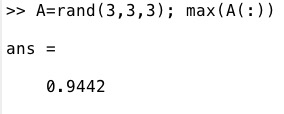% maxmax(A) - returns the maximal maximum of a multidimensional matrix% (by Tim Lueth, VLFL-Lib, 2017-JUN-05 as class: AUXILIARY PROCEDURES)% % =======================================================================% OBSOLETE (2017-06-05) - USE FAMILY 'max(D(:))' INSTEAD% =======================================================================% % See also: [ max(D(:)) ] ; maxmax, minmin% % M=maxmax(A)% === INPUT PARAMETERS ===% A: matrix% === OUTPUT RESULTS ======% M: largest maximum of A% % EXAMPLE: % maxmax(rand(2,2,2))%
###### minmin(A)- returns the minimal minum of a multidimensional matrix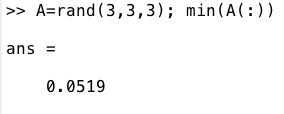% minmin(A) - returns the minimal minum of a multidimensional matrix% (by Tim Lueth, VLFL-Lib, 2017-JUN-05 as class: AUXILIARY PROCEDURES)% % =======================================================================% OBSOLETE (2017-06-05) - USE FAMILY 'min(D(:))' INSTEAD% =======================================================================% % See also: [ min(D(:)) ] ; maxmax% % M=minmin(A)% === INPUT PARAMETERS ===% A: matrix% === OUTPUT RESULTS ======% M: min(min(.....% % EXAMPLE: % minmin(rand(2,2,2))%
###### removeimat(D,r,c)- removes a row and a column of a indexed matrix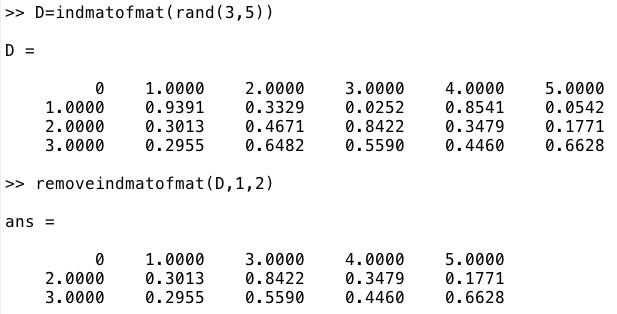% removeimat(D,r,c) - removes a row and a column of a indexed matrix% (by Tim Lueth, VLFL-Lib, 2017-JUN-05 as class: AUXILIARY PROCEDURES)% % See also: imat% % ND=removeimat(D,r,c)% === INPUT PARAMETERS ===% D: indexed matrix% r: row to delete% c: column to delete% === OUTPUT RESULTS ======% ND: Matrix without this column and row% % EXAMPLE: % D=imat(rand(3,5)), removeimat(D,1,2)%
###### imat(D)- returns a matrix including row column and column row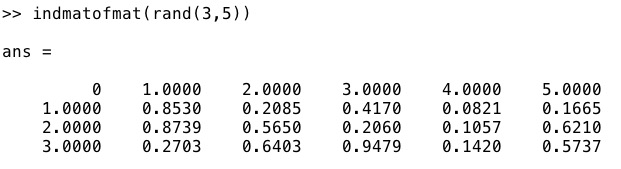% imat(D) - returns a matrix including row column and column row% (by Tim Lueth, VLFL-Lib, 2017-JUN-05 as class: AUXILIARY PROCEDURES)% % See also: imat, removeimat% % DN=imat(D)% === INPUT PARAMETERS ===% D: matrix 2x2% === OUTPUT RESULTS ======% DN: matrix plus row column and column row% % EXAMPLE: % D=imat(rand(3,5))%
###### textCVL (CVL,c,s,nt,lb)- plots descriptors for each contour into a figure using text command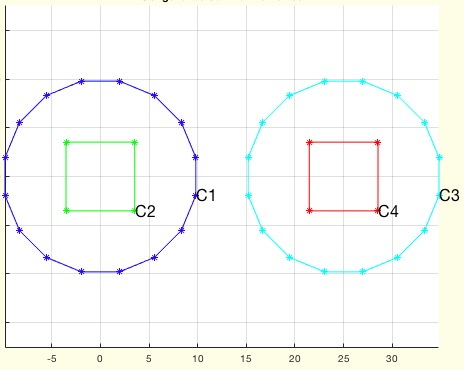% textCVL (CVL,c,s,nt,lb) - plots descriptors for each contour into a figure using text command% (by Tim Lueth, VLFL-Lib, 2017-JUN-05 as class: VISUALIZATION)% % up to 99 contours are marked at the first point by a letter and a % number (Status of: 2017-06-05)% % See also: textT, textP, textVL% % textCVL(CVL,[c,s,nt,lb])% === INPUT PARAMETERS ===% CVL: Contour list separated by Nan (CPL/CVL)% c: Color, default is black% s: Size, default is 16% nt: optional list of selected contours% lb: optional string for text; default is 'C'% % EXAMPLE: Different examples for plotting% SGfigure; CPL=CPLsample(12); CVLplot(CPL); textCVL(CPL);%
###### nearestpair(PLA,PLB,tp);- returns the nearest point pairs of two vector list% nearestpair(PLA,PLB,tp); - returns the nearest point pairs of two vector list% (by Tim Lueth, VLFL-Lib, 2017-JUN-04 as class: ANALYTICAL GEOMETRY)% % Two vector list are processed using the norm distance. If the parameter % 'unique' is used, then e vector that was used already cannot be used a % second time. The index zero shows that there is no corresponding vector % in the second list (Status of: 2017-06-18)% % See also: nearestcluster, connectofmat, norm, PLcorrelate, CPLcorrelate% % [p,x,D]=nearestpair(PLA,PLB,[tp]);% === INPUT PARAMETERS ===% PLA: Vector list A% PLB: Vector list B% tp: 'min', 'mean' ; default is 'min'% === OUTPUT RESULTS ======% p: index in B for each point of A% x: Distance between the pairs% D: Full Distance matrix% % EXAMPLE: % D1=rand(10,2); D2=rand(10,2); % nearestpair(D1,D2,'mean');% nearestpair(D1,D2,'min');%
###### circshiftCPL(CPL,n)- returns a circular shifted CPL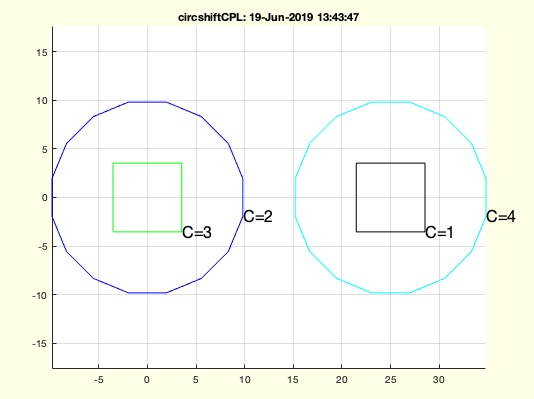% circshiftCPL(CPL,n) - returns a circular shifted CPL% (by Tim Lueth, VLFL-Lib, 2017-JUN-04 as class: CLOSED POLYGON LISTS)% % This is more or less for testing CPLcorrelate (Status of: 2017-06-04)% % See also: CPLcircshift% % CPL=circshiftCPL(CPL,[n])% === INPUT PARAMETERS ===% CPL: Closed Polygon List% n: number of circshifts polygon; default is random% === OUTPUT RESULTS ======% CPL: resulting CPL% % EXAMPLE: % SGfigure; B=circshiftCPL(CPLsample(13)) ;CVLplot(circshiftCPL(B));% SGfigure; B=circshiftCPL(CPLsample(14)) ;CVLplot(circshiftCPL(B));%
###### CPLcorrelate(CPLA,CPLB)- correlates the contours from two CPLs% CPLcorrelate(CPLA,CPLB) - correlates the contours from two CPLs% (by Tim Lueth, VLFL-Lib, 2017-JUN-04 as class: CLOSED POLYGON LISTS)% % In contrast to PLcorrelate, which correlates points for a single pair % of a single contour, this fnctn here correlates contours to preprare % the use of PLcorrelate. The correlation List containts in each row the % corresponding contours of A and B, the level of the contour (0=most % outside) and the parent contour of Ai and the parent contour of Bi% The parameter list constains:% the row number, the parent, the level and the center point (Status of: % 2017-06-07)% % See also: FLofPLcorrelation, PLcorrelate, textCVL% % [CLL,a,b]=CPLcorrelate(CPLA,CPLB)% === INPUT PARAMETERS ===% CPLA: Contour A% CPLB: Contour B% === OUTPUT RESULTS ======% CLL: Correlation list [ACi BCi Level PAi PBi]% a: complete list of used parameter of a% b: complete list of used parameter of a% % EXAMPLE: % CPLcorrelate(CPLsample(26),CPLsample(27))% CPLcorrelate(CPLsample(27),CPLsample(26))%
###### modN(n,k,N)- returns mod function for elements N:N+k(-1)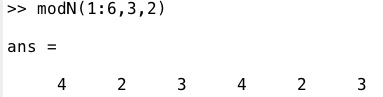% modN(n,k,N) - returns mod fnctn for elements N:N+k(-1) % (by Tim Lueth, VLFL-Lib, 2017-JUN-04 as class: AUXILIARY PROCEDURES)% % auxiliary fnctn for array manipulation. Instead of return values % between [0..k-1], this fnctn returns [N..N+k-1] by a=mod(n-N,k)+N % (Status of: 2017-06-04)% % See also: mod1, mod% % a=modN(n,k,[N])% === INPUT PARAMETERS ===% n: number% k: divider% N: Interval start% === OUTPUT RESULTS ======% a: rest% % EXAMPLE: % modN(1:6,3)% modN(1:6,3,2)%
###### FLofPLcorrelation(CL,offset,VL)- returns a facet list for a given point correspondance list% FLofPLcorrelation(CL,offset,VL) - returns a facet list for a given point correspondance list% (by Tim Lueth, VLFL-Lib, 2017-JUN-02 as class: SURFACES)% % See also: PLcorrelate% % FL=FLofPLcorrelation(CL,[offset,VL])% === INPUT PARAMETERS ===% CL: Correspondance list% offset: % VL: optional vertex list for a drawing% === OUTPUT RESULTS ======% FL: Facet list created by the correspondance list% % EXAMPLE: % [CL,VL]=PLcorrelate(CPLsample(3),PLcircle(10,4));% FLofPLcorrelation(CL,'',VL);%
###### CPLconvexseg(CPL)- returns the segments of convex and concave contours a CPL.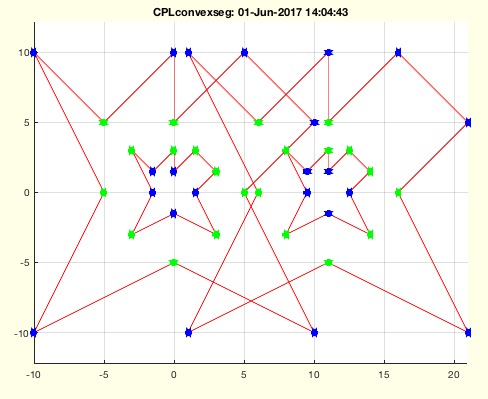% CPLconvexseg(CPL) - returns the segments of convex and concave contours a CPL.% (by Tim Lueth, VLFL-Lib, 2017-JUN-01 as class: ANALYTICAL GEOMETRY)% % ATTENTION. Convex and concave are related to ccw orientation% +1 = convex (for ccw) [= concave for cw]% -1 = concave (for ccw) [= convex for cw]% Same fnctn as PLconvexseg for NAN separated CPLs (Status of: 2017-06-01)% % See also: isconvexPL, PLconvexseg% % con=CPLconvexseg(CPL)% === INPUT PARAMETERS ===% CPL: Single Point List% === OUTPUT RESULTS ======% con: array of convex and concave% % EXAMPLE: % CPLconvexseg(PLcircle(10,6))% CPLconvexseg(flipud(PLcircle(10,6)))% PLconvexseg(PLsample(9))%
###### PLconvexseg(PL)- returns the segments of convex and concave conoturs within ONE CPL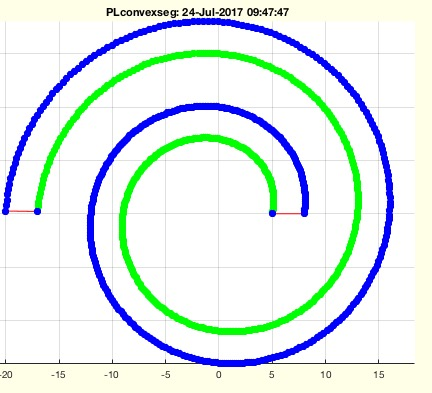% PLconvexseg(PL) - returns the segments of convex and concave conoturs within ONE CPL % (by Tim Lueth, VLFL-Lib, 2017-JUN-01 as class: ANALYTICAL GEOMETRY)% % ATTENTION. Convex and concave are related to ccw orientation% +1 = convex (for ccw) [= concave for cw]% -1 = concave (for ccw) [= convex for cw]% Same fnctn as CPLconvexseg for single PL (Status of: 2017-07-24)% % Introduced first in SolidGeometry 3.9% % See also: isconvexPL, CPLconvexseg, CPLisccwcorrected% % [con,AL,CIL]=PLconvexseg(PL)% === INPUT PARAMETERS ===% PL: Single Point List% === OUTPUT RESULTS ======% con: array of convex and concave% AL: Angle List% CIL: Change index list plus status [n x 3]% % EXAMPLE: % PLconvexseg(CPLsample(3));% PLconvexseg(PLsample(9));% PLconvexseg(CPLisccwcorrected(PLsample(9)));% % See also: isconvexPL, CPLconvexseg, CPLisccwcorrected% % % Copyright 2017 Tim C. Lueth
###### replacemat(A,valold,valnew)- replaces values in vectors and matrices% replacemat(A,valold,valnew) - replaces values in vectors and matrices% (by Tim Lueth, VLFL-Lib, 2017-JUN-01 as class: AUXILIARY PROCEDURES)% % mainly: A(A==valold(i))=valnew(i)% tab(mat) (Status of: 2017-06-01)% % replacemat(A,valold,valnew)% === INPUT PARAMETERS ===% A: vectors or matrices% valold: existing values% valnew: new values% % EXAMPLE: % A=[1 2 3; 4 5 6], replacemat(A,1,9)% A=[1 2 3; 4 5 6], replacemat(A,[1 9],[9 0])%
###### distofintervall(a,b,inter)- distance within an intervall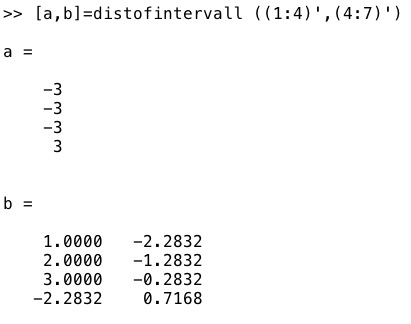% distofintervall(a,b,inter) - distance within an intervall% (by Tim Lueth, VLFL-Lib, 2017-MAI-31 as class: AUXILIARY PROCEDURES)% % maps a value in circular intervall (Status of: 2017-05-31)% % See also: norm% % [d,iv]=distofintervall(a,b,[inter])% === INPUT PARAMETERS ===% a: value 1 % b: value 2% inter: intervall; default is [-pi +pi]% === OUTPUT RESULTS ======% d: distance ()% iv: value a in intervall% % EXAMPLE: % a=distofintervall (-pi+pi/10,pi-pi/10)% a=distofintervall (1:10,2:11)%
###### PLminangle(PL,in0,C,at)- returns the point with minimal angle value wrt to the center of the contour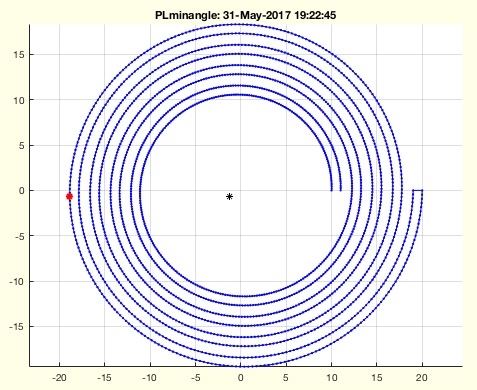% PLminangle(PL,in0,C,at) - returns the point with minimal angle value wrt to the center of the contour% (by Tim Lueth, VLFL-Lib, 2017-MAI-31 as class: AUXILIARY PROCEDURES)% % See also: PLminyx% % [P,pin,AL,DL,C]=PLminangle(PL,[in0,C,at])% === INPUT PARAMETERS ===% PL: Point Liste% in0: false=[-pi..+pi]; true=[0..2*pi]; default is false% C: optional center point% at: angle threshold; default is pi/100% === OUTPUT RESULTS ======% P: Point with minimal y and minimal x% pin: find index in PL% AL: Angle list% DL: Distance from Center List% C: Center Point% % EXAMPLE: % PLminangle(CPLspiral(10,20,8*pi))% PLminabgle(CPLspiral(10,20,8*pi),true)% PLminangle(CPLspiral(10,20,8*pi),true,[0 0])% [~,ci]=PLminyx(CPLB); CPLB=circshift(CPLB,-(ci-1));%
###### PLcorrelate(PLA,PLB,stype,ctype,mtype)- returns the correlation of two single point lists% PLcorrelate(PLA,PLB,stype,ctype,mtype) - returns the correlation of two single point lists% (by Tim Lueth, VLFL-Lib, 2017-MAI-31 as class: CLOSED POLYGON LISTS)% % Auxiliary fnctn to correlate two point lists. It was designed first in % SGof2CPLz.% SGof2CPLz is still more powerful. (Status of: 2017-06-04)% % See also: SGof2CPLz, FLofPLcorrelation, CPLcorrelate% % [CL,VL]=PLcorrelate([PLA,PLB,stype,ctype,mtype])% === INPUT PARAMETERS ===% PLA: Point list A% PLB: Point list B% stype: 'number','length','angle','center','nearest', default 'center'% ctype: 'org','minx','mina'/'rot'; default is 'mina'% mtype: 'none', 'norm', 'norm1'% === OUTPUT RESULTS ======% CL: Correlation List% VL: Vertex list [PLA;PLAB] without doubled points % % EXAMPLE: % PLcorrelate(CPLsample(3),circshift(PLofCPL(CPLsample(3)),1))% PLcorrelate(PLcircle(15),CPLsample(3))%
###### SGofCPLzchamfer(CPL,z,ph,ed);- returns a solid with chamfered edges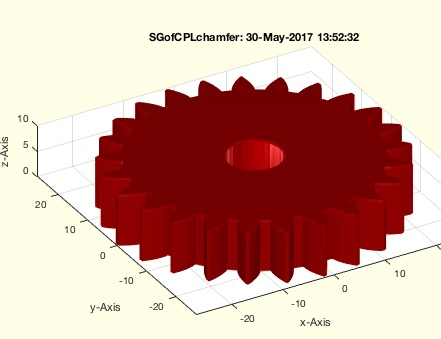% SGofCPLzchamfer(CPL,z,ph,ed); - returns a solid with chamfered edges% (by Tim Lueth, VLFL-Lib, 2017-MAI-30 as class: SURFACES)% % Based on a comment of Florian Schleich. The body is divided into three % planes. In order to ensure the calculation of the walls, the % orientations of the contour are first adapted. (Status of: 2017-05-30)% % See also: SGofCPLz, SGof2CPLsz% % SG=SGofCPLzchamfer([CPL,z,ph,ed]);% === INPUT PARAMETERS ===% CPL: Closed Polygon line% z: Height z% ph: phase default is 0.3% ed: curved edges; default is true% === OUTPUT RESULTS ======% SG: Resulting solid% % EXAMPLE: % SGofCPLchamfer([CPLsample(21);NaN NaN;PLcircle(5)],10)% SGofCPLzchamfer% %
###### CPLisccwcorrected(CPL)- returns a CPL with all CPLs in correct orientation cw/ccw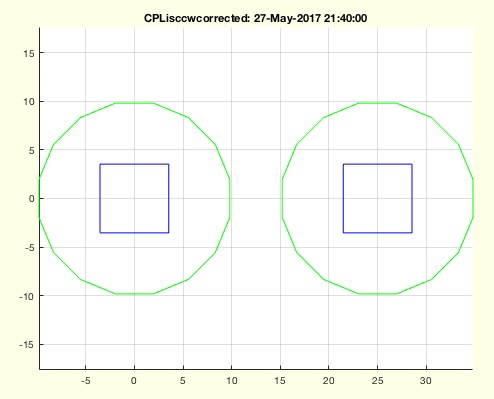% CPLisccwcorrected(CPL) - returns a CPL with all CPLs in correct orientation cw/ccw% (by Tim Lueth, VLFL-Lib, 2017-MAI-27 as class: CLOSED POLYGON LISTS)% % See also: CPLisccw, CPLsortinout, CPLisccwinout% % [CPL,cio]=CPLisccwcorrected([CPL])% === INPUT PARAMETERS ===% CPL: Original CPL% === OUTPUT RESULTS ======% CPL: Corrected CPL% cio: inside outside index%
###### CPLisccwinout(CPL)- returns which contour has the right orientation wrt shell and orientation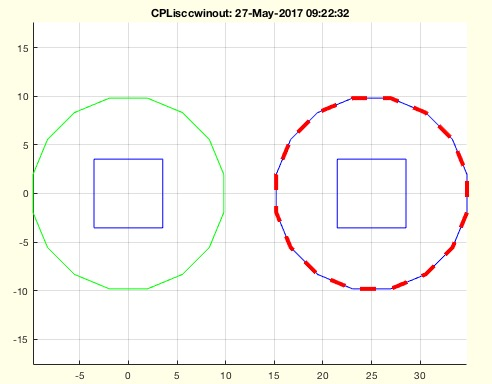% CPLisccwinout(CPL) - returns which contour has the right orientation wrt shell and orientation% (by Tim Lueth, VLFL-Lib, 2017-MAI-27 as class: CLOSED POLYGON LISTS)% % Depending on the outwards level, the orientation of a contour is:% 0 = outward = ccw% 1 = inward = cw% 2 = outward = ccw% 3 .....% if length(corr)==sum(corr); the contour is correct (Status of: % 2017-05-27)% % See also: CPLisccw, CPLsortinout, CPLisccwcorrected% % [corr,cio,ccw]=CPLisccwinout(CPL)% === INPUT PARAMETERS ===% CPL: Contour polygon list% === OUTPUT RESULTS ======% corr: true if correct% cio: level of contour (0=most outwards)% ccw: ccw direction% % EXAMPLE: % FCPL=replaceNaN(CPLsample(12),3,flipud(separateNaN(CPLsample(12),3)));% CPLisccwinout(FCPL)% CPLisccwinout(CPLsample(13))%
###### replaceNaN(CPL,ci,CPLi)- replaces a contour within a CPL% replaceNaN(CPL,ci,CPLi) - replaces a contour within a CPL% (by Tim Lueth, VLFL-Lib, 2017-MAI-27 as class: CLOSED POLYGON LISTS)% % Introduced first in SolidGeometry 3.9% % See also: selectNaN, separateNaN, cellofNaN% % CPL=replaceNaN(CPL,ci,CPLi)% === INPUT PARAMETERS ===% CPL: CPL% ci: index of contour% CPLi: new contour for contour ci% === OUTPUT RESULTS ======% CPL: % % EXAMPLE: % replaceNaN(CPLsample(12),3,flipud(separateNaN(CPLsample(12),3)));% % See also: selectNaN, separateNaN, cellofNaN%
###### CVLseparatez(CVL,z,thr)- returns a sliced CVL/CPL for a given z value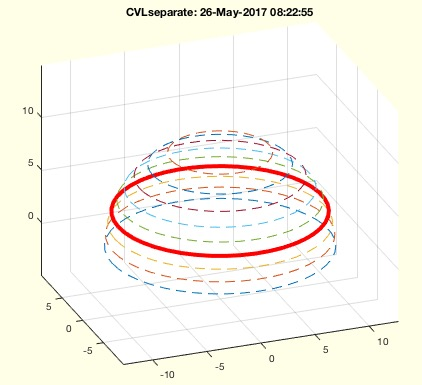% CVLseparatez(CVL,z,thr) - returns a sliced CVL/CPL for a given z value% (by Tim Lueth, VLFL-Lib, 2017-MAI-26 as class: CLOSED POLYGON LISTS)% % for sliced CVL, i.e. CPL with an added z value, this fnctn returns only % the contours that have the same z value (Status of: 2017-07-23)% % Introduced first in SolidGeometry 3.9% % See also: CVLofSGslices, SGofCVLslices% % [CVLz,zL]=CVLseparatez(CVL,[z,thr])% === INPUT PARAMETERS ===% CVL: Slices CVL% z: desired z value; currently only scalar% thr: tolerance for finding a z value% === OUTPUT RESULTS ======% CVLz: Contours that fulfill the z-value condition; separated by NaN OR % zl if isempty(z)% zL: List of z Values% % EXAMPLE: % CVL=CVLofSGslices(SGsample(5),10);% CVLseparatez(CVL,3.3333333);% % See also: CVLofSGslices, SGofCVLslices% % % Copyright 2017 Tim C. Lueth